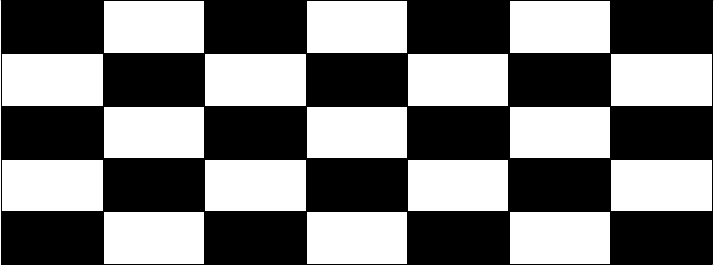⭐️引言⭐️

这里是不会变成少年的恶龙，如果你也喜欢刷题，那我们就是好朋友啦！近期在更新蓝桥杯模拟题题解，模拟题相对真题来说难度小很多，非常适合小白当入门基础训练。不断刷题不断进步，让我们一起加油！（个人时间精力有限，没有办法一次性将题解写的很完美，后续有时间会不断改进更正）

个人题解，仅供参考学习，有错误麻烦私信更正啦！

A.128的倍数

B.特殊年份

C.奇不动排列

D.上楼梯

E.递增三元组

F.路程

G.棋盘染色问题

H.最大停车位

I.人字排列

J.教学楼

# A.128的倍数

请问在10000 (含) 到90000 (含)中, 有多少个数是128的倍数。

这是一道结果填空的题，你只需要算出结果后提交即可。本题的结果为一个整数，在提交答案时只填写这个整数，填多余的内容将无法得分。

``````ans = 0                           #定义变量记录结果
for i in range(10000,90000,128):  #遍历范围，间隔为128
ans +=1
print(ans)                        #输出625``````

# B.特殊年份

问题描述

2021是一个特殊的年份，它的千位和十位相同，个位比百位多一。请问从1000 (含)到9999 (含)有多少个这样的年份?

这是一道结果填空的题，你只需要算出结果后提交即可。本题的结果为一个整数,在提交答案时只填写这个整数,填写多余的内容将无法得分。

暴力枚举就行，我做的算复杂了，优不优化意义不大，填空题能算出答案就行。

``````ans = 0                               #定义全局变量记录结果
for i in range(1000,10000):           #循环
a = str(i)                        #将数值型转化为字符串
if (a==a) and (int(a)==int(a)-1):  #条件判断
ans +=1
print(ans)                            #输出81``````

# C.奇不动排列

如果1到n的一个排列中，所有奇数都在原来的位置上,称为一个奇不动排列。

例如，1到7的排列(1,6,3,4,5,2, 7)是一一个奇不动排列，因为1, 3, 5, 7都在原来的位置上。

请问，1到21的所有排列中，有多少个奇不动排列。

这是一道结果填空的题，你只需要算出结果后提交即可。本题的结果为一个整数，在提交答案时只填写这个整数，填写多余的内容将无法得分。

很简单的全排列问题，介绍一下全排列：

``````ans = 1
for i in range(10,1,-1): #直接循环，正反都一样
ans =ans*i
print(ans)               #输出3628800
``````

# D.上楼梯

可以口算出结果最大是5，上楼梯的顺序是1,5,5,4,-1,-9。

# E.递增三元组

在数列a, a, ... a[n]中,如果对于下标ij, k满足0<i<j<k<n+1且a[i<a[j]<a[k], 则称aij, a[j], a[k]为一组递增三元组。

请问对于下面的长度为20的数列，有多少个递增三元组?

2, 9, 17,4, 14, 10, 25, 26, 11,14, 16, 17, 14,21, 16, 27, 32, 20, 26, 36。

这是一道结果填空的题，你只需要算出结果后提交即可。本题的结果为一个整数，在提交答案时只填写这个整数,填写多余的内容将无法得分。

这题数据量不大可以直接用三层循环枚举出来。

``````L=input().split(",")              #输入

L=[int(i) for i in L]             #将字符串转换成整型进行操作
ans = 0                           #定义全局变量记录结果

for i in range(21):                #三层循环遍历范围
for j in range(i,21):
for z in range(j,20):
if L[i] < L[j] < L[z]: #判断条件
ans +=1

print(ans)                         #输出565
``````

# F.路程

小蓝开车在高速上行驶了t小时，速度固定为每小时v千米,请问小蓝在高速上行驶了多长路程?

输入一行包合两个整数t、v, 用一个空格分隔。

输出一行包含一个整数,表示答案。

3 81

243

对于所有评测用例，1 <=t<= 10, 1 <=v<= 150。

``````n,m=map(int,input().split())
ans=n*m
print(ans)
``````

# G.棋盘染色问题

问题描述

有一个n * m的棋盘,现在对这个棋盘进行黑白染色,左上角染成黑色。从左上角开始，每个黑色格的相邻格染成白色，白色格的相邻格染成黑色。

以下给出了一个5*7的棋盘的染色示例。

给定n和m,请问棋盘上一共有多少方格被染成了色。

输入一行包含两个整数n, m,用一个空格分隔。

输出一行包含一个整数，表示答案。

5 7

18根据图形我们可以发现：奇数行的奇数列都是黑色方块，偶数行的偶数列都是黑色方块。

``````n,m = map(int,input().split())
a = len([int(i) for i in range(1,m+1,2)])
b = len([int(i) for i in range(2,m,2)])
num = 0
for i in range(1,n+1):
if i%2 !=0:
num +=a
else:
num +=b
print(num)
``````

将棋盘每两行分为一组，发现每组中黑色格子占每组总格子数的一半。

假设棋盘为 m 行 n 列
如果 m 为偶数，答案是所有格子数的一半,即num =  m * n / 2
如果 m 为奇数，num = (m - 1) * n / 2 + (n + 1) / 2 = (m * n + 1) / 2
当 m 为偶数时答案等价于num =  (m * n + 1) / 2

``````​n,m = map(int,input().split())
ans = (m*n +1)/2
print(ans)
``````

# H.最大停车位

小蓝要在路边划分停车位。他将路边可停车的区域划分为L个整数小块,编号1至L。一个车位需要连续的k个小块，停车位不能重叠。有的小块属于井盖、消防通道等区域，不能停车。请问小蓝最多划分出多少个停车位?

输入的第一行包含三个整数L、k、n,分别表示小块数量。一个车位需要的小块数量和不能停车的小块数量,相邻整数之间用一个空格分隔。

第二行包含n个整数a, a, ... a[n],按从小到大的顺序排列，相邻的整数间用空格分隔，示这些位置不能停车。

输出一行包含一个整数, 表示答案。

100 10 2

25 91

8

题目也很简单，直接上代码（笔者精力有限无法一时将分析和代码写的很详细，后续会慢慢更正的）：

``````L,k,n = map(int,input().split())
num = list(map(int,input().split()))
a = len(num)
ans = 0
for i in range(a):
if i==a-1:
break
ans +=(num[i+1] - num[i])//k

ans = num//k + (L-num[a-1])//k + ans
print(ans)
``````

# I.人字排列

一个1到n的排列被称为人字排列，是指排列中的第1到第(n+1)/2个元愫单调递增，第(n+1)/2到第n元素单调递减。

例如: (2,3,5,8,9,7,6,4,1)是一个人字排列，而(1, 2,3)和(2, 1, 3)都不是人字排列，(2, 4, 3)也不是一个人字排列(它甚至不是一个1到4的排列)。

请问，1到n的排列中有多少个人字排列?

输入一行包含一个奇数n。

输出一行包含一个整数, 示答案,答案可能很大,请输出答案除以100000007的余数。

5

6

# J.教学楼

问题描述

著名设计师小蓝给蓝桥小学设计了一个教学楼。

输入的第一行包含两个整数n, m,用一个空格分隔。

接下来n行，每行一个长度为m的01串,示教学楼的平面图。

如果满足要求，输出"YES” ，否则输出"NO”,请注意母全部都是大写。